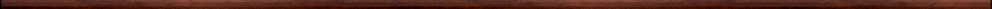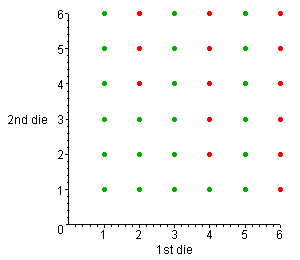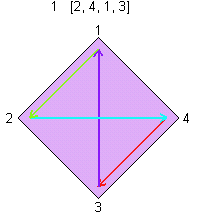Maple worksheets on sets, counting and probabilityElementary mathematics topics:

They are all compatible with Classic Worksheet Maple 10.An introduction to counting methods - count.mws

• The multiplication principle
• Arrangement of n things in a line
• General permutations of r objects taken from n
• Selections or combinations

An introduction to probability by simulation using random numbers - prob.mws

• Random numbers using: rand and rand(n..m)
• Simulation of flipping a coin: flipcoin.
• Rolling two dice and adding the two numbers
• Sample spaces for probability experiments
• Events and definition of probability
• More experiments with two dice

Sets and the addition formula for probabilities - sets.mws

• Sets
• The union and intersection of sets
• Complementation
• Disjoint sets and mutually exclusive events
• The addition formula for probabilities
• An example involving rolling two diceComplete permutations - cpltperm.mws

• The letterbox problem - simple cases
• An inductive formula for the number L(k) of complete permutations
• A simpler inductive formula for L(k)
• The limiting value of the probability of a complete permutation
• Simulations for the experimental probability of complete permutations
• The inclusion-exclusion principle
• Using the inclusion-exclusion principle to obtain a formula for L(k)
• A procedure for constructing pictorial representations of complete permutationsTop of page

Main index